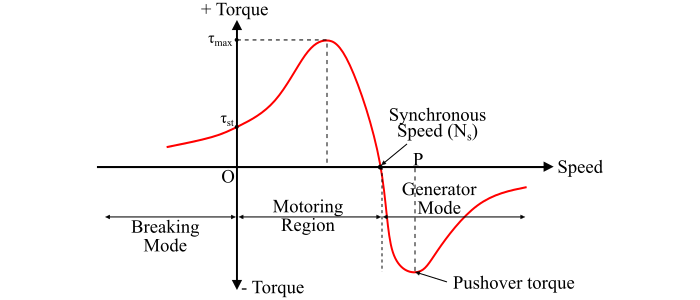# Induction Generator (Asynchronous Generator)

Sometimes, a 3-phase induction machine is used as a generator. Initially, the induction machine is started as a motor and it draws lagging reactive power from the supply mains. Then, the speed of the machine is increased above the synchronous speed by an external prime mover in the same direction as the rotating magnetic field of the stator so that the induction machine will now operate as an induction generator and will produce a generating torque.

The direction of this generating torque is opposite to the rotation of the rotor. Hence, under these conditions, the slip in the machine is negative and the induction generator delivers electrical power to the supply mains. The complete torque-speed characteristic of a 3-phase induction machine for all speed ranges is shown in the figure.The equivalent circuit of the induction generator is shown in the figure below.Here, the mechanical shaft load has been replaced by a resistor whose value is given by,

$$\mathrm{𝑅_𝑚 =\frac{𝑅_2}{𝑠}(1 − 𝑠)}$$

Where, R2 is the rotor circuit resistance.

As we know, in an induction generator, the slip s is negative and hence, the load resistance (Rm) equivalent to mechanical shaft load is also negative, which shows that the load resistance does not absorb power, but acts as a source of power, i.e., the induction generator delivers electrical power to the supply mains to which it is connected.

The output of the induction generator depends upon the magnitude of the negative slip.

From the torque-speed characteristic of the induction machine, it can be seen that there is a maximum possible torque in the generating mode which is called as pushover torque of the generator. If the torque applied by the prime mover is greater than the pushover torque, then the induction generator will over-speed.

Since the rotating magnetic field in an induction machine is produced by the exciting current which is supplied to the stator windings from the supply mains. Hence, an induction generator is not a self-excited generator. Therefore, it is necessary to excite the stator of the machine with an external source at all times at the rated voltage and frequency and driven at a speed above the synchronous speed. As it can be noted that the speed of the induction generator is different from the synchronous speed, hence, it is also known as asynchronous generator.

Following are primary advantages of an induction generator −

• The induction generator has a robust construction and hence requires less maintenance.
• The induction generators are less expensive.
• A squirrel cage induction can be used as an induction generator.
• The size per kW output power of an induction generator is relatively small.
• Synchronism with the supply line is not required for the operation of the induction generator.
• The induction generator requires less number of auxiliary equipment.
• When a short-circuit fault occurs on the terminals of the machine, the excitation fails and the machine stops generation. Therefore, the induction generator has a self-protective feature.
• The induction generator runs in parallel without hunting.
• The variation in the speed of the external prime mover driving the induction generator is less important.